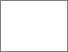A source-extraction based coupling method for computational aeroacoustics

Wang, Zongkang (2004) A source-extraction based coupling method for computational aeroacoustics. PhD thesis, University of Greenwich.Preview
PDF (Pages containing signatures redacted)
Zongkang Wang 2004 - redacted.pdf - Published Version

Abstract

This thesis involves the computation of aerodynamically generated sound using a source-extraction based coupling approach.

In the present coupling method, the unsteady aerodynamic calculation and the calculation of sound propagation are separated artificially. A set of acoustic perturbation equations is derived by decomposing all flow variables into their dominant part and their fluctuating part, and neglecting some small-magnitude terms, and further simplified into a set of isentropic perturbation equations. Accompanying the derivation of the acoustic perturbation equations, a new extracting formulation for the acoustic source terms contained in the unsteady flow field is proposed. The acoustic source terms required in solving the acoustic perturbation equations are computed numerically from the time-dependent solutions of the unsteady flow field.

In the simulation of the unsteady flow, the unsteady Reynolds-Averaged-Navier-Stokes equations (RANS) based cell-centred finite volume method is mainly used. A large eddy simulation (LES) technique is also employed in the investigation of one application case. A powerful and efficient high order dispersion-relation-preserving (DRP) finite difference scheme with fully staggered-grid variable arrangements is implemented in the solution of the acoustic perturbation equations. The performance of a set of radiation boundary conditions is examined for various background flows. A suitable and efficient coupling procedure, in conjunction with the source-extraction formulation, is designed between the cell-centred finite volume based CFD solver and the fully-staggered finite difference based acoustic solver.

A range of acoustic model problems are investigated with the purpose of assessing the feasibility and accuracy of the source-extraction formulation associated with the coupling procedure. These model problems include wave propagation, reflection, interaction, and scattering, of acoustic pulse with/without background mean flow. The accuracy of computational results from these model problems is very encouraging when reasonable computational mesh sizes and time steps are used in both the CFD solver and the acoustic solver.

Several applications of the source-extraction based coupling method to some more complex cases have also been examined. These cases are: 1) generation and propagation of sound by a series of vortices impinging on a finite thin flat plate; 2) generation and propagation of sound from a subsonic flow past a finite thin flat plate with a small angle of attack; 3) generation and near field radiation of aerodynamic sound from an low speed, laminar flow over a two-dimensional automobile door cavity; 4) flow-induced noise from an open cavity turbulent flow. These application calculations have demonstrated preliminarily the capability and potential of the new source extraction formulation for solving more realistic aeroacoustic problems.

Item Type: Thesis (PhD) uk.bl.ethos.401563 computational aeroacoustics, equations Q Science > QA MathematicsT Technology > TL Motor vehicles. Aeronautics. Astronautics School of Computing & Mathematical SciencesSchool of Computing & Mathematical Sciences > Department of Mathematical Sciences 13 Feb 2019 10:11 None None None None None http://gala.gre.ac.uk/id/eprint/6339View Item